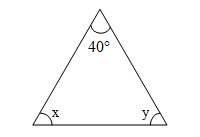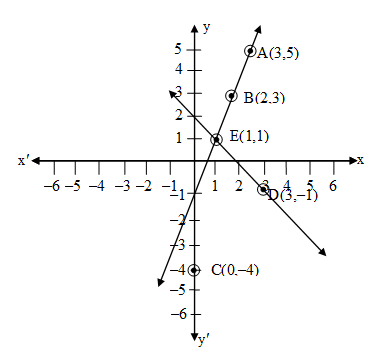Filter By

## All Questions

#### The angles of a cyclic quadrilateral ABCD are A = (6x + 10)°, B = (5x)° C = (x + y)°, D = (3y – 10)° Find x and y, and hence the values of the four angles.

Solution:
According to property of cyclic quadrilateral, sum of opposite angles = .
A + C =
6x +  + x + y =
7x + y =
7x + y =               … (1)
Similarly B + D =
5x + 3y – =
5x + 3y = +
5x + 3y =            … (2)
Solving eq. (1) and eq. (2) we get
21x + 3y =  {Multiply eq. (1) by 3}        … (3)
Now subtract equation (2) from (3) then we get
16x =

x =
Put x = in eq. (1) we get
7() + y =
y =
y =
Hence A = 6x +
= 6 ×  +
= +
=
B = (5x)
= 5 ×  =

( + ) =
D = (3y – 10)°
= 3 ×

=
Hence the value of four angles are:
, and  respectively.

#### In a competitive examination, one mark is awarded for each correct answer while mark is deducted for every wrong answer. Jayanti answered 120 questions and got 90 marks. How many questions did she answer correctly?

Solution:
Let x be the number of correct answer and (120 – x) be the number of wrong answer .
According to question

x –

3x – 120 = 180
3x = 180 + 120
3x = 300

x = 100
Hence Jayanti answered 100 questions correctly.

## Crack CUET with india's "Best Teachers"

• HD Video Lectures
• Unlimited Mock Tests
• Faculty Support#### A shopkeeper gives books on rent for reading. She takes a fixed charge for the first two days, and an additional charge for each day thereafter. Latika paid Rs 22 for a book kept for six days, while Anand paid Rs 16 for the book kept for four days. Find the fixed charges and the charge for each extra day.

Solution:
Let x be the fixed charges and y be the extra charges for each day.
According to question equation for latika is
x + 4y = 22                  … (1)
For Anand
x + 2y = 16                  … (2)
Subtract equation (1) and (2) we get
4y – 2y = 22 – 16
2y = 6

y =  = 3
Put y = 3 in equation (1)
x + 4 (3) = 22
x = 22 – 12
x = 10
The fixed charge is = 10 Rs.

#### There are some students in the two examination halls A and B. To make the number of students equal in each hall, 10 students are sent from A to B. But if 20 students are sent from B to A, the number of students in A becomes double the number of students in B. Find the number of students in the two halls.

Solution:
According to question:
Let x and y are the number of students in class A and B.
x – 10 = y + 10
x – y = 20                    … (1)
Second condition is
(x + 20) = 2(y – 20)
x + 20 = 2y – 40
x – 2y = – 60               … (2)
Subtract (1) and (2) we get
y = 20 + 60
y = 80
Put y = 80 in (1)
x – 80 = 20
x = 20 + 80
x = 100
Hence in hall A there are 100 students and in hall B there are 80 students.

## Crack NEET with "AI Coach"

• HD Video Lectures
• Unlimited Mock Tests
• Faculty Support#### Two numbers are in the ratio 5:6. If 8 is subtracted from each of the numbers, the ratio becomes 4:5. Find the numbers

Solution:
Let x and y be two numbers
According to question
x : y = 5 : 6

x =             … (1)
According to second condition

5x – 40 = 4y – 32
5x – 4y = –32 + 40
5x – 4y = 8      … (2)
Put value of x from equation (1) in (2) we get

25y – 24y = 48
y = 48
Put y = 48 in eq. (1)
x =
x = 40

#### The age of the father is twice the sum of the ages of his two children. After 20years, his age will be equal to the sum of the ages of his children. Find the age of the father.

Solution:
Let x be the age of father y and z be the ages of children
According to the question:
x = 2 (y + z)                … (1)
After 20 years
x + 20 = (y + 20) + (z + 20)
x + 20 = y + z + 40
x + 20  – 40 = y + z
x – 20 = y + z              … (2)
Put the value of (y + z) from equation (2) in equation (1) we get
x = 2 (x – 20)
x = 2x – 40
x – 2x = – 40
–x = – 40
x = 40
Hence father’s age is 40 years

## Crack JEE Main with "AI Coach"

• HD Video Lectures
• Unlimited Mock Tests
• Faculty Support#### Two years ago, Salim was thrice as old as his daughter and six years later, he will be four years older than twice her age. How old are they now?

Solution: Let  x is the age of salim
y is the age of his daughter
According to question
(x – 2) = 3(y – 2)
x – 2 = 3y – 6
x – 3y = –4 …(1)
According to second condition
x + 6 = 2(y + 6) + 4
x + 6 = 2y + 12 + 4
x – 2y = 10 … (2)
Solve (1) and (2) by substitution method
x – 3y = – 4
x – 2y = 10
–    +       –
–y = – 14
y = 14
Put y = 14 in equation (1)
x – 3(14) = – 4
x – 42 = –4
x = –4 + 42
x = 38
Salim’s age = 38 years
His daughter’s age = 14 years

#### The angles of a triangle are x, y and . The difference between the two angles x and y is . Find x and y.

Solution:
Given: x – y =  …(1)According to sum of interior angles of a triangle’ property
x + y +  = 180
x + y =  … (2)
Solve eq. (1) and eq. (2) using substitution method.
x – y =
x + y =
2x = 170

x =
x =
Put x = in eq. (1) we get
– y =
= y
= y

## Crack CUET with india's "Best Teachers"

• HD Video Lectures
• Unlimited Mock Tests
• Faculty Support#### If x + 1 is a factor of 2x3+ ax2+ 2bx + 1, then find the values of a and b given that 2a – 3b = 4.

Solution:

It is given that (x + 1) is a factor of P(x) = 2x3 + ax2 + 2bx + 1 then according to remainder theorem P(–1) is equal to 0.

P(–1) = 2(–1)3 + a(–1)2 + 2b (–1) + 1
0 = –2 + a – 2b + 1
a – 2b = 1 … (1)
Given 2a – 3b = 4 … (2)
Solving eq. (1) and (2) by substitution method
2a – 4b = 2                  {multiply eq. (1) by 2}
2a – 3b = 4
–     +     –
–b = –2
b = 2
Put b = 2 in equation (1)
a – 2(2) = 1
a – 4 = 1
a = 1 + 4
a = 5

#### Write an equation of a line passing through the point representing solution of the pair of linear equations x + y = 2 and 2x – y = 1. How many such lines can we find?

Solution:
Given equations are    x + y = 2
2x – y = 1
x + y = 2

 x 1 2 3 y 1 0 -1

2x – y = 1

 x 1 2 3 y 1 3 5From the graph it is clear that two lines AB and CD intersect and point E (1, 1) Hence infinite lines can pass through the intersecting point E like 4 = x, 2x – y = 1 etc.